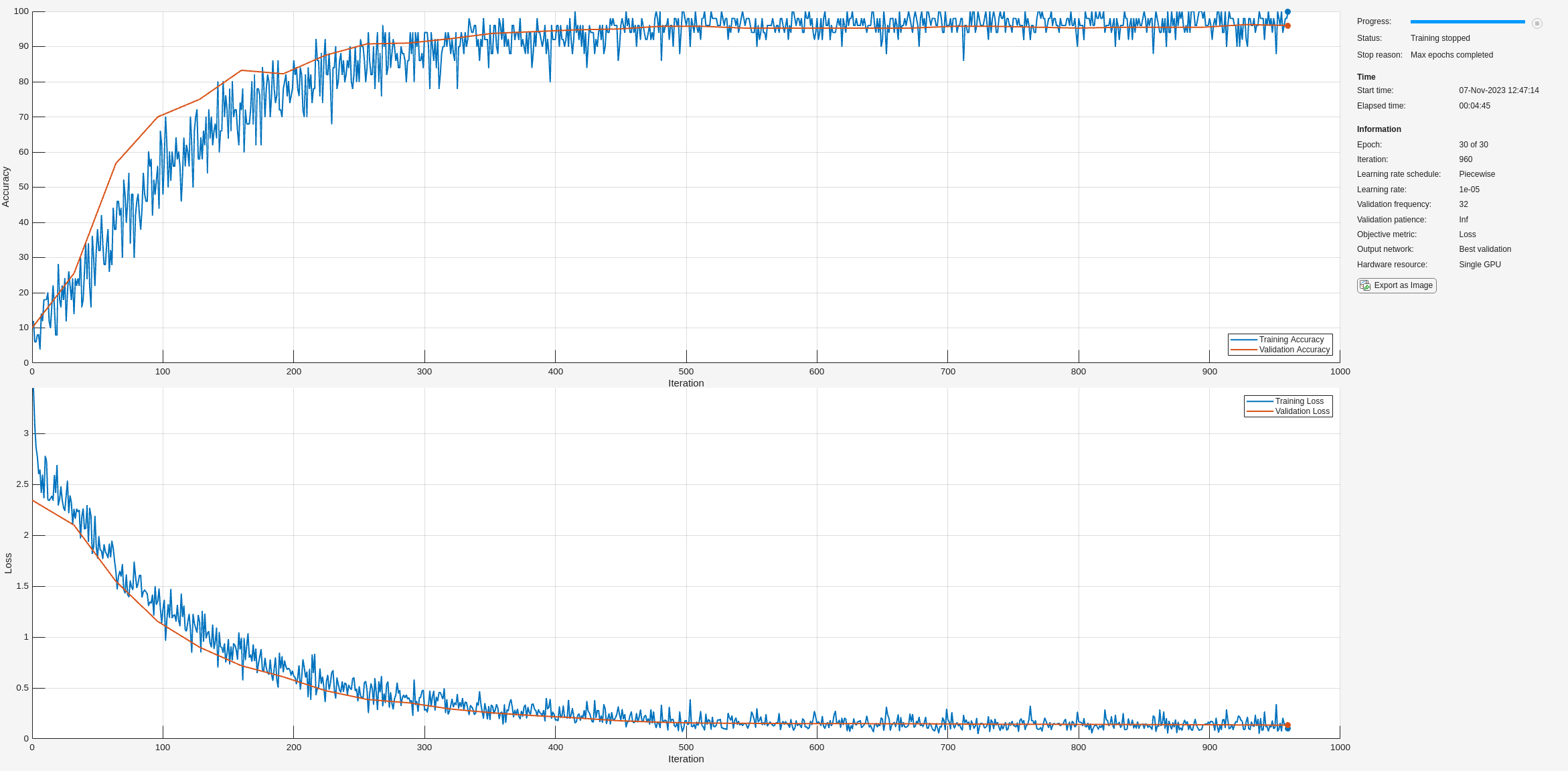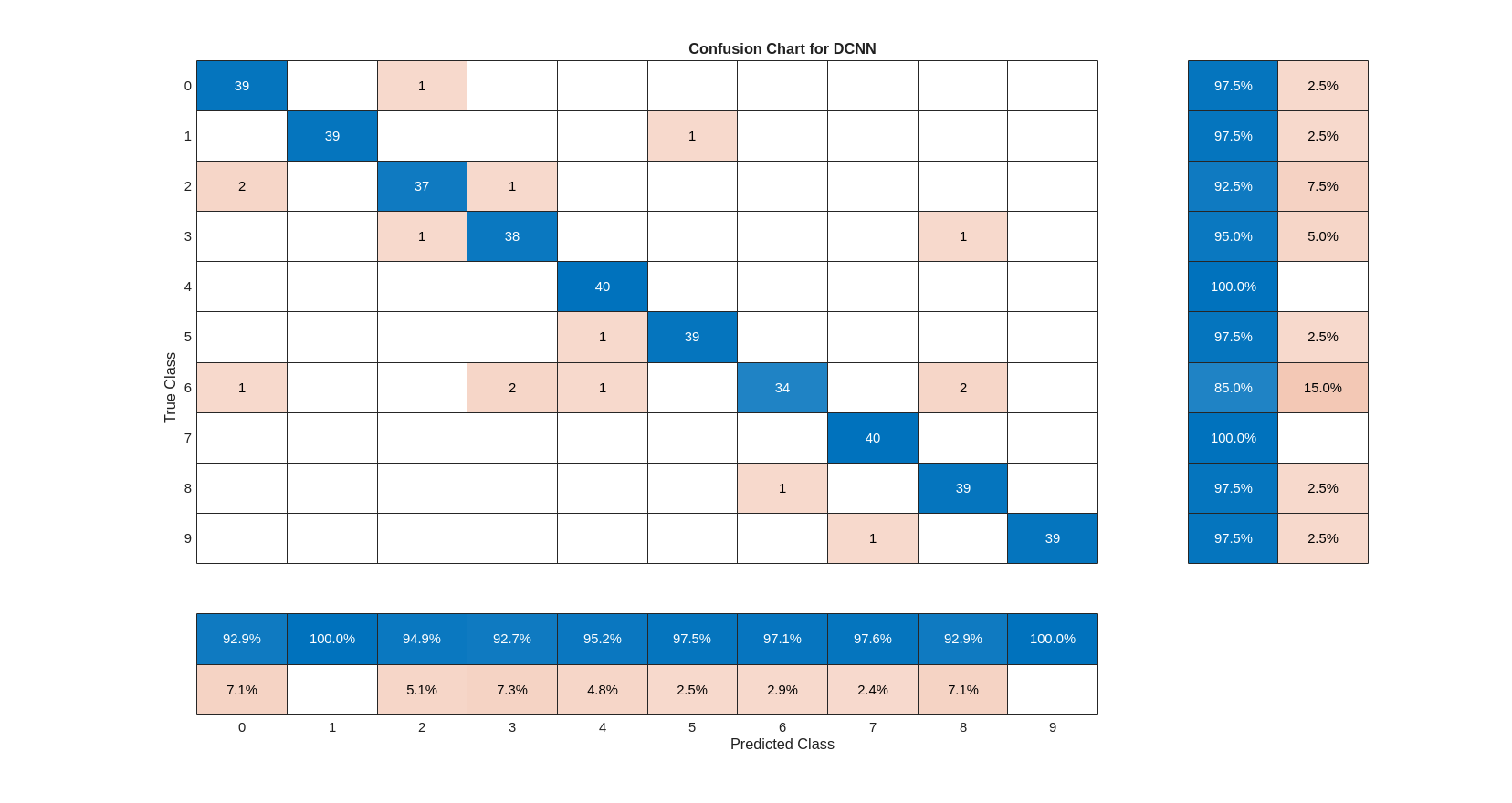# Train Spoken Digit Recognition Network Using Out-of-Memory Features

This example trains a spoken digit recognition network on out-of-memory auditory spectrograms using a transformed datastore. In this example, you extract auditory spectrograms from audio using `audioDatastore` (Audio Toolbox) and `audioFeatureExtractor` (Audio Toolbox), and you write them to disk. You then use a `signalDatastore` to access the features during training. The workflow is useful when the training features do not fit in memory. In this workflow, you only extract features once, which speeds up your workflow if you are iterating on the deep learning model design.

### Data

Download the Free Spoken Digit Data Set (FSDD). FSDD consists of 2000 recordings of four speakers saying the numbers 0 through 9 in English.

```downloadFolder = matlab.internal.examples.downloadSupportFile("audio","FSDD.zip"); dataFolder = tempdir; unzip(downloadFolder,dataFolder) dataset = fullfile(dataFolder,"FSDD");```

Create an `audioDatastore` that points to the dataset.

`ads = audioDatastore(dataset,IncludeSubfolders=true);`

Display the classes and the number of examples in each class.

```[~,filenames] = fileparts(ads.Files); ads.Labels = categorical(extractBefore(filenames,'_')); summary(ads.Labels)```
``` 0 200 1 200 2 200 3 200 4 200 5 200 6 200 7 200 8 200 9 200 ```

Split the FSDD into training and test sets. Allocate 80% of the data to the training set and retain 20% for the test set. You use the training set to train the model and the test set to validate the trained model.

```rng default ads = shuffle(ads); [adsTrain,adsTest] = splitEachLabel(ads,0.8); countEachLabel(adsTrain)```
```ans=10×2 table Label Count _____ _____ 0 160 1 160 2 160 3 160 4 160 5 160 6 160 7 160 8 160 9 160 ```
`countEachLabel(adsTest)`
```ans=10×2 table Label Count _____ _____ 0 40 1 40 2 40 3 40 4 40 5 40 6 40 7 40 8 40 9 40 ```

### Reduce Training Dataset

To train the network with the entire dataset and achieve the highest possible accuracy, set `speedupExample` to `false`. To run this example quickly, set `speedupExample` to `true`.

```speedupExample =false; if speedupExample adsTrain = splitEachLabel(adsTrain,2); adsTest = splitEachLabel(adsTest,2); end```

### Set up Auditory Spectrogram Extraction

The CNN accepts mel-frequency spectrograms.

Define parameters used to extract mel-frequency spectrograms. Use 220 ms windows with 10 ms hops between windows. Use a 2048-point DFT and 40 frequency bands.

```fs = 8000; frameDuration = 0.22; frameLength = round(frameDuration*fs); hopDuration = 0.01; hopLength = round(hopDuration*fs); segmentLength = 8192; numBands = 40; fftLength = 2048;```

Create an `audioFeatureExtractor` (Audio Toolbox) object to compute mel-frequency spectrograms from input audio signals.

```afe = audioFeatureExtractor(melSpectrum=true,SampleRate=fs, ... Window=hamming(frameLength,"periodic"),OverlapLength=frameLength - hopLength, ... FFTLength=fftLength);```

Set the parameters for the mel-frequency spectrogram.

`setExtractorParameters(afe,"melSpectrum",NumBands=numBands,FrequencyRange=[50 fs/2],WindowNormalization=true);`

Create a transformed datastore that computes mel-frequency spectrograms from audio data. The supporting function, `getSpeechSpectrogram`, standardizes the recording length and normalizes the amplitude of the audio input. `getSpeechSpectrogram` uses the `audioFeatureExtractor` object `afe` to obtain the log-based mel-frequency spectrograms.

`adsSpecTrain = transform(adsTrain,@(x)getSpeechSpectrogram(x,afe,segmentLength));`

### Write Auditory Spectrograms to Disk

Use `writeall` (Audio Toolbox) to write auditory spectrograms to disk. Set `UseParallel` to true to perform writing in parallel.

```outputLocation = fullfile(tempdir,"FSDD_Features"); writeall(adsSpecTrain,outputLocation,WriteFcn=@myCustomWriter,UseParallel=true);```

### Set up Training Signal Datastore

Create a `signalDatastore` that points to the out-of-memory features. The read function returns a spectrogram/label pair.

```sds = signalDatastore(outputLocation,IncludeSubfolders=true, ... SignalVariableNames=["spec","label"],ReadOutputOrientation="row");```

### Validation Data

The validation dataset fits into memory. Precompute validation features.

```adsTestT = transform(adsTest,@(x){getSpeechSpectrogram(x,afe,segmentLength)}); XTest = readall(adsTestT); XTest = cat(4,XTest{:});```

Get the validation labels.

`YTest = adsTest.Labels;`

### Define CNN Architecture

Construct a small CNN as an array of layers. Use convolutional and batch normalization layers, and downsample the feature maps using max pooling layers. To reduce the possibility of the network memorizing specific features of the training data, add a small amount of dropout to the input to the last fully connected layer.

```sz = size(XTest); specSize = sz(1:2); imageSize = [specSize 1]; numClasses = numel(categories(YTest)); dropoutProb = 0.2; numF = 12; layers = [ imageInputLayer(imageSize,Normalization="none") convolution2dLayer(5,numF,Padding="same") batchNormalizationLayer reluLayer maxPooling2dLayer(3,Stride=2,Padding="same") convolution2dLayer(3,2*numF,Padding="same") batchNormalizationLayer reluLayer maxPooling2dLayer(3,Stride=2,Padding="same") convolution2dLayer(3,4*numF,Padding="same") batchNormalizationLayer reluLayer maxPooling2dLayer(3,Stride=2,Padding="same") convolution2dLayer(3,4*numF,Padding="same") batchNormalizationLayer reluLayer convolution2dLayer(3,4*numF,Padding="same") batchNormalizationLayer reluLayer maxPooling2dLayer(2) dropoutLayer(dropoutProb) fullyConnectedLayer(numClasses) softmaxLayer classificationLayer(Classes=categories(YTest)); ];```

Set the hyperparameters to use in training the network. Use a mini-batch size of 50 and a learning rate of 1e-4. Specify '`adam`' optimization. To use the parallel pool to read the transformed datastore, set `DispatchInBackground` to `true`. For more information, see `trainingOptions` (Deep Learning Toolbox).

```miniBatchSize = 50; options = trainingOptions("adam", ... InitialLearnRate=1e-4, ... MaxEpochs=30, ... LearnRateSchedule="piecewise", ... LearnRateDropFactor=0.1, ... LearnRateDropPeriod=15, ... MiniBatchSize=miniBatchSize, ... Shuffle="every-epoch", ... Plots="training-progress", ... Verbose=false, ... ValidationData={XTest,YTest}, ... ValidationFrequency=ceil(numel(adsTrain.Files)/miniBatchSize), ... ExecutionEnvironment="auto", ... DispatchInBackground=true);```

Train the network by passing the training datastore to `trainNetwork`.

`trainedNet = trainNetwork(sds,layers,options);`Use the trained network to predict the digit labels for the test set.

```[Ypredicted,probs] = classify(trainedNet,XTest); cnnAccuracy = sum(Ypredicted==YTest)/numel(YTest)*100```
```cnnAccuracy = 96 ```

Summarize the performance of the trained network on the test set with a confusion chart. Display the precision and recall for each class by using column and row summaries. The table at the bottom of the confusion chart shows the precision values. The table to the right of the confusion chart shows the recall values.

```figure(Units="normalized",Position=[0.2 0.2 1.5 1.5]); confusionchart(YTest,Ypredicted, ... Title="Confusion Chart for DCNN", ... ColumnSummary="column-normalized",RowSummary="row-normalized");```### Supporting Functions

#### Get Speech Spectrograms

```function X = getSpeechSpectrogram(x,afe,segmentLength) % getSpeechSpectrogram(x,afe,params) computes a speech spectrogram for the % signal x using the audioFeatureExtractor afe. x = scaleAndResize(single(x),segmentLength); spec = extract(afe,x).'; X = log10(spec + 1e-6); end```

#### Scale and Resize

```function x = scaleAndResize(x,segmentLength) % scaleAndResize(x,segmentLength) scales x by its max absolute value and forces % its length to be segmentLength by trimming or zero-padding. L = segmentLength; N = size(x,1); if N > L x = x(1:L,:); elseif N < L pad = L - N; prepad = floor(pad/2); postpad = ceil(pad/2); x = [zeros(prepad,1);x;zeros(postpad,1)]; end x = x./max(abs(x)); end```

#### Custom Write Function

```function myCustomWriter(spec,writeInfo,~) % myCustomWriter(spec,writeInfo,~) writes an auditory spectrogram/label % pair to MAT files. filename = strrep(writeInfo.SuggestedOutputName,".wav",".mat"); label = writeInfo.ReadInfo.Label; save(filename,"label","spec"); end```